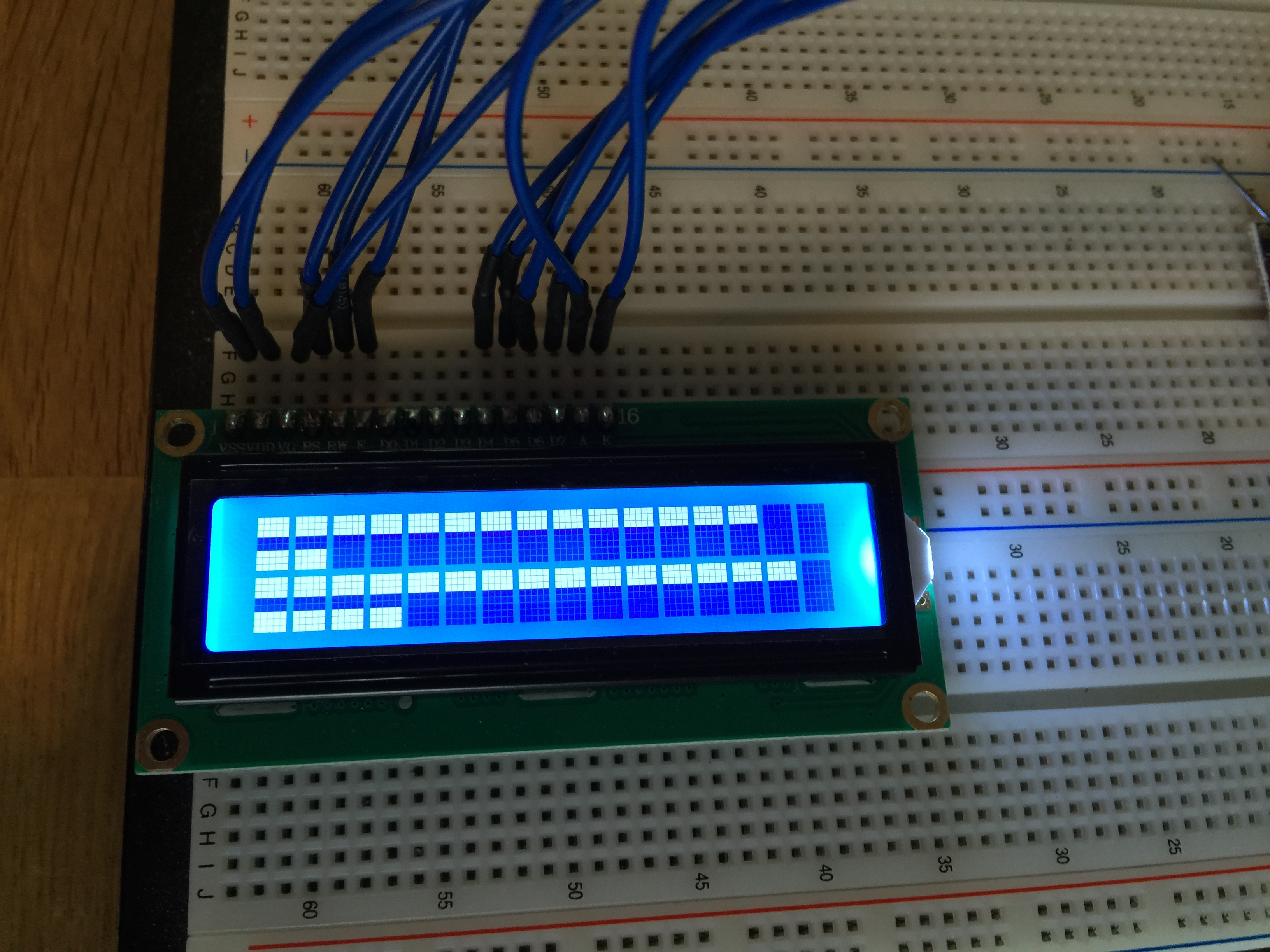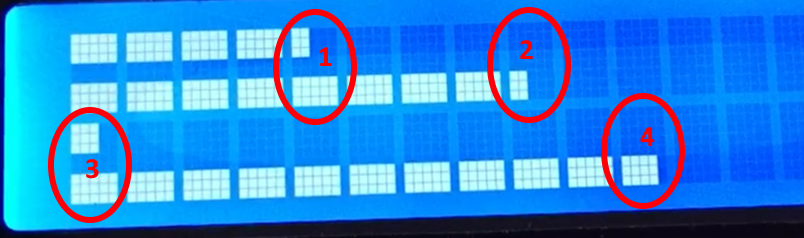# HD44780 – Create Multiple BargraphsThis article is about how to use multiple detailed bargraphs at the same time on one HD44780 LC-Display. I focus on the coding. The wiring depends on your display board and setup. If you do not know how to connect your display, check google. This code uses this HD44780 library.

The HD44780 controller has no built in function for creating detailed bargraphs, because you can only display characters that are stored in the CGROM of the controller. These characters depend on the version of the controller, most time you have the “European standard font” version. Check the datasheet for detailed information for your own controller. You can download the datasheet at the end of this page.

### But how to create a bargraph?

The trick is to use the integrated CGRAM of the controller. CGRAM is a storage in the HD44780 for user generated chars. I can hold up to 8 custom made characters.

For bargraphs, these 3 characters are always the same. They can be stored in the CGRAM at the startup of the application.

To store these 3 custom chars in the ROM of the controller I made this small function:

```void lcd_add_static_chars() {
uint8_t char10 = {0b11111, 0b11111, 0b11111, 0b00000, 0b00000, 0b00000, 0b00000, 0b00000},// first row
char01 = {0b00000, 0b00000, 0b00000, 0b00000, 0b00000, 0b11111, 0b11111, 0b11111},// seconds row
char11 = {0b11111, 0b11111, 0b11111, 0b00000, 0b00000, 0b11111, 0b11111, 0b11111};// both
lcd_generatechar(5, char10);
lcd_generatechar(6, char01);
lcd_generatechar(7, char11);
}```

Until now you are able to display bargraphs with just these 3 characters. The resolution will be 16 steps from 0% to 100% with a 2×16 display. So you get steps of 6.25%.

For some projects this might be enough, but for a higher resolution you should use every pixel column inside every character. This would bring a possible resolution of 16 * 5 steps from 0% to 100%, which equals 1.25% stepsize. This is very accurate for a bargraph display!

To achieve this, the ending of every bargraph must be generated “on the fly”. When displaying 4 different bargraphs at the same time, there are 4 different possible end characters.Since we used 3 chars of the CGRAM, there are 5 more available. So there is enough storage available for our 4 auto generated characters.

I created a small algorithm for generating custom characters on the fly, which is integrated in the function lcd_display_bars.

• The argument “*tmpValues” is the array of values (0 = 0%; 80 = 100%)
• Argument “rows” is the number of rows used on the LCD (1 = 2 bargraphs; 2 = 4 bargraphs)
```void lcd_display_bars(uint8_t *tmpValues, uint8_t rows) {
uint8_t endChars = {0}, currentEndChar = 0;
for(uint8_t lcd_row = 0; lcd_row < rows; lcd_row++) {
for(uint8_t i = 1; i <= 16; i++) { if((tmpValues[0 + 2 * lcd_row] > (i - 1) * 5 && tmpValues[0 + 2 * lcd_row] < i * 5) || tmpValues[1 + 2 * lcd_row] > (i - 1) * 5 && tmpValues[1 + 2 * lcd_row] < i * 5) {
for(uint8_t c = 0; c < 6; c++) { if(tmpValues[0 + 2 * lcd_row] >= ((i - 1) * 5) + c)
for(uint8_t r = 0; r < 3; r++)
endChars[currentEndChar][r] |= (1 << 4 - c + 1); if(tmpValues[1 + 2 * lcd_row] >= ((i - 1) * 5) + c)
for(uint8_t r = 5; r < 8; r++)
endChars[currentEndChar][r] |= (1 << 4 - c + 1); } lcd_generatechar(currentEndChar, endChars[currentEndChar]); lcd_setcursor(i - 1, lcd_row + 1); lcd_data(currentEndChar); currentEndChar++; } else { lcd_setcursor(i - 1, lcd_row + 1); if(tmpValues[0 + 2 * lcd_row] >= i * 5 && tmpValues[1 + 2 * lcd_row] >= i * 5) lcd_data(uint8_t(7));
else if(tmpValues[0 + 2 * lcd_row] >= i * 5 && tmpValues[1 + 2 * lcd_row] < i * 5) lcd_data(uint8_t(5));
else if(tmpValues[0 + 2 * lcd_row] < i * 5 && tmpValues[1 + 2 * lcd_row] >= i * 5) lcd_data(uint8_t(6));
else lcd_data(' ');
}
}
}
}```

### Demo code

This demo code updates 4 bargraphs every 2 seconds with random gerenerated values.

```#include
#include "lcd-routines.h"
#include "detailed-bargraphs.h"

int main(void)
{
lcd_init(); // init display
uint8_t values;

while(1)
{
for(uint8_t i = 0; i < 4; i++) {
values[i] = rand() % (5 * 16); // generate random values
}

lcd_display_bars(&values, 2); // update bargraphs

_delay_ms(2000); // wait 2 seconds
}
}```#### About the Author: Hauke Marquardt

Student Elektrotechnik & Informatik

1.Karri says:
2.ted says: